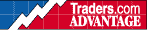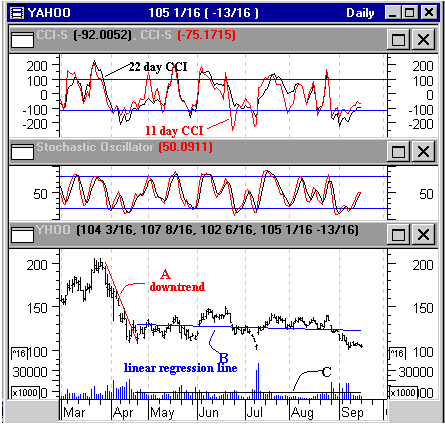HOT TOPICS LIST

INDICATORS LIST

LIST OF TOPICS

# Commodity Channel Index

09/19/00 02:46:41 PM
by Dennis D. Peterson

The Commodity Channel Index can be a useful alternative to stochastics.

Security:   YHOO
Position:   N/A

 The Commodity Channel Index (CCI) is an oscillator developed by Donald Lambert in 1980. It is based on the notion that as an equity tops or bottoms the volatility decreases. Lambert was struck by the fact that an equity can resemble a sine wave as it moves between highs and lows. The observation is obviously most valid when an equity is in a price channel. The CCI takes advantage of cycles -- if the trader can see a cycle then CCI can be "tuned" to fit into it. CCI is calculated using the following steps:1. Calculate the typical price:Typical price = (high + low + close)/3.2. Calculate the simple moving average of the typical price for n number of periods. 3. Subtract the moving average of the typical price for n days from the typical price for each day.4. Calculate mean absolute deviation as the sum of absolute value differences of:Typical price (nth day) - moving average of typical price for n days, going back n days, and dividing by the number of days. 5. CCI is (today's typical price - moving average of typical price) / 0.015 x (mean absolute deviation).What this all means is that if today's typical price exceeds the average deviation of typical price then we have a positive number, and if an equity is breaking out then this could be a substantial number. Certainly this is a way to help identify a breakout.CCI relates to stochastics because they are both looking at how price reacts to range. CCI is a measure of volatility. The closer the analyst can see a cycle, the more powerful CCI will be. If you pick n as number that is too big you stand the risk of incorporating cycles that are going to have CCI with a mean absolute deviation that is so large, CCI is almost always less than 100. If n is too small then CCI will be exceeding 100 too often.Figure 1: CCI versus stochastics for Yahoo. CCI (upper chart) uses 11-day and 22-day periods to look for intermediate trends. Stochastics (middle chart) use 5 for %K, then a 3-period moving average for slow, and 3 for %D. Yahoo (bottom chart) appears to have an 11-day cycle. Buy/sell signals for CCI work better than stochastics (middle chart). Graphic provided by: MetaStock. For this example I choose Yahoo (YHOO:Nasdaq) (Figure 1). First, determine when Yahoo is not trending. The downtrend (A in Figure 1) was broken in mid-April and Yahoo started its trend channel. A linear regression line (B in Figure 1) through Yahoo was an easy way to confirm the trend was nearly horizontal. I also wanted the volume to be reasonably level as well, and this is confirmed with the horizontal linear regression line (C in Figure 1). What can be observed is Yahoo seems to be in an up and down cycle every 15 calendar days or so, and so the cycle is about 11 trading days. To obtain intermediate cycles, I went with 11-day rather than 5-day cycles for a short term indicator. One other twist is that CCI can be used like a stochastic. I plot 11-day and 22-day cycles (upper Chart: Figure 1) and look for crossovers, the same way you would with a stochastic. I also color coded the two CCI's the same way as the stochastic (middle chart: Figure 1) using red for fast and black for slow. Using the CCI crossovers as buy and sell signals work better than the stochastic. You could argue the right choice of stochastic should give the same result. But how would you choose the right stochastic parameters? CCI at least gives you a shot by looking at the data. The first breakout in June is predicted as well as the second one in July.

Dennis D. Peterson

Market index trading on a daily basis.

 Comments or Questions? Article Usefulness 5 (most useful) 4 3 2 1 (least useful)

Date:�/ /Rank:�4Comment:
Date:�/ /Rank:�5Comment: